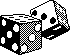dice-play How to do...

The Three Dice Guessing Trick

An effective trick requiring some simple mathematics and three dice.  Pick a subject and give them the three dice, and a pen and paper or calculator if their mental arithmetic isn't up to scratch.  Ask them to roll the three dice so that you can't see the resulting numbers (with your back to them or with a throwing cup to cover the dice).  Then ask your subject to follow the simple steps listed below so that you can miraculously guess what the numbers thrown are.  For example, if they have thrown a 3, 6, and 2 they...

 Multiply the number on the first die by 2 6 Add 5 11 Multiply by 5 55 Add the number on the second die - 6 61 Multiply by 10 610 Add the number on the third die - 2 612 Subtract 125 487

All you have to do is ask for the resulting number and subtract 125 from it.  This gives you a three digit value with each digit representing a number thrown on the dice. i.e. 487 - 125 = 362 or 3 - 6 - 2.  Try and make this last step seem difficult, as if you are using some magical power.